# An AI for 2048 — Part 1

So I played the game 2048 and thought it would be fun to create an AI for the game.  I wrote my code in Mathematica because it is a fun, terse language with easy access to numerical libraries since I was thinking some simple linear or logit regression ought to give me some easy to code strategies.

The game itself is pretty easy to code in Mathematica.  The game is played on a 4×4 grid of tiles.  Each tile in the grid can contain either a blank (I will hereafter use a _ to denote a blank), or one of the numbers 2,4,8,16,32,64,128, 256, 512, 1024, or 2048.  On every turn, you can shift the board right, left, up, or down. When you shift the board, any adjacent matching numbers will combine.

The object of the game is to get one 2048 tile which usually requires around a thousand turns.

Here’s an example.  Suppose you have the board below.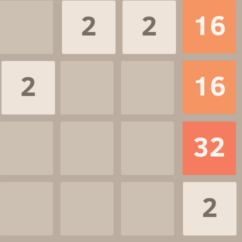If you shift right, the top row will become _, _, 4, 16, because the two twos will combine.  Nothing else will combine.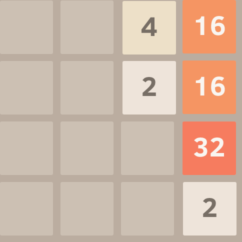Shifting left gives the same result as shifting right except all of the tiles are on the left side instead of the right.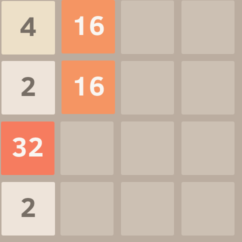If we look back at the original image (below), we can see that there are two sixteen tiles stacked vertically.So the original board (above) shifted down combines the two sixteens into a thirty-two (below) setting some possible follow-up moves like combining the twos on the bottom row or combining the two thirty-twos into a sixty-four.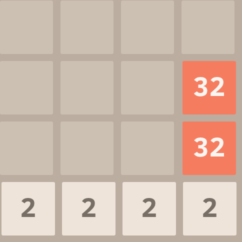There are a few other game features that make things more difficult.  After every move, if the board changes, then a two or four tile randomly fills an empty space. So, the sum of the tiles increases by two or four every move.

## Random Play

The first strategy I tried was random play.  A typical random play board will look something like this after 50 moves.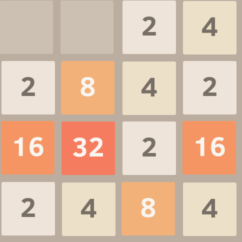If you play for a while, you will think that this position is in a little difficult because the high numbers are not clumped, so they might be hard to combine.  A typical random game ends in a position like this one.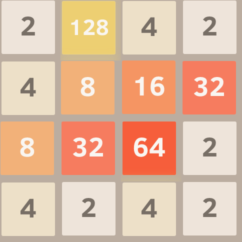In the board above, the random player is in deep trouble.  His large tiles are near the center, the 128 is not beside the 64, there are two 32 tiles separated by the 64, and the board is almost filled.  The player can only move up or down.  In this game the random player incorrectly moved up combining the two twos in the lower right and the new two tile appeared in the lower right corner square ending the game.

I ran a 1000 random games and found that the random player ends the game with an average tile total of 252.  Here is a histogram of the resulting tile totals at the end of all 1000 games.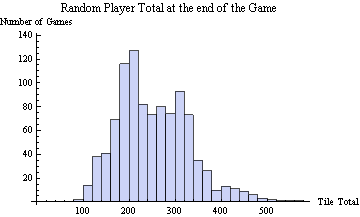(The random player’s tile total was between 200 and 220 one hundred and twenty seven times.  He was between 400 and 420 thirteen times.)

The random player’s largest tile was 256 5% of the time, 128 44% of the time, 64 42% of the time, 32 8% of the time, and 16 0.5% of the time.  It seems almost impossible to have a max of 16 at the end.  Here is how the random player achieved this ignominious result.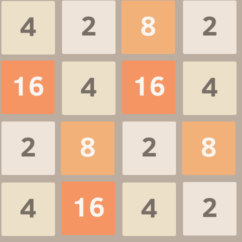## The Greedy Strategy

So what’s a simple improvement?  About the simplest possible improvement is to try to score the most points every turn—the greedy strategy.  You get the most points by making the largest tile possible.  In the board below, the player has the options of going left or right combining the 32 tiles, or moving up or down combining the two 2 tiles in the lower left.

The greedy strategy would randomly choose between left or right combining the two 32 tiles.  The greedy action avoids splitting the 32 tiles.

I ran 1000 greedy player games games.  The average tile total at the end of a greedy player simulation was 399—almost a 60% improvement over random play. Here is a histogram of the greedy player’s results.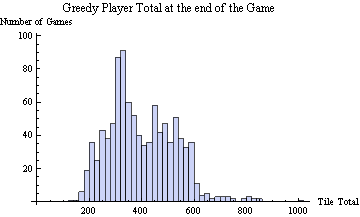If you look closely, you will see that is a tiny bump just above 1000.  In all 1000 games, the greedy player did manage to get a total above 1000 once, but never got the elusive 1024 tile.  He got the 512 tile 2% of the time, the 256 tile 43% of the time, the 128 44%, the 64 11%, and in three games, his maximum tile was 32.

I ran a number of other simple strategies before moving onto the complex look ahead strategies like UCT.  More on that in my next post.